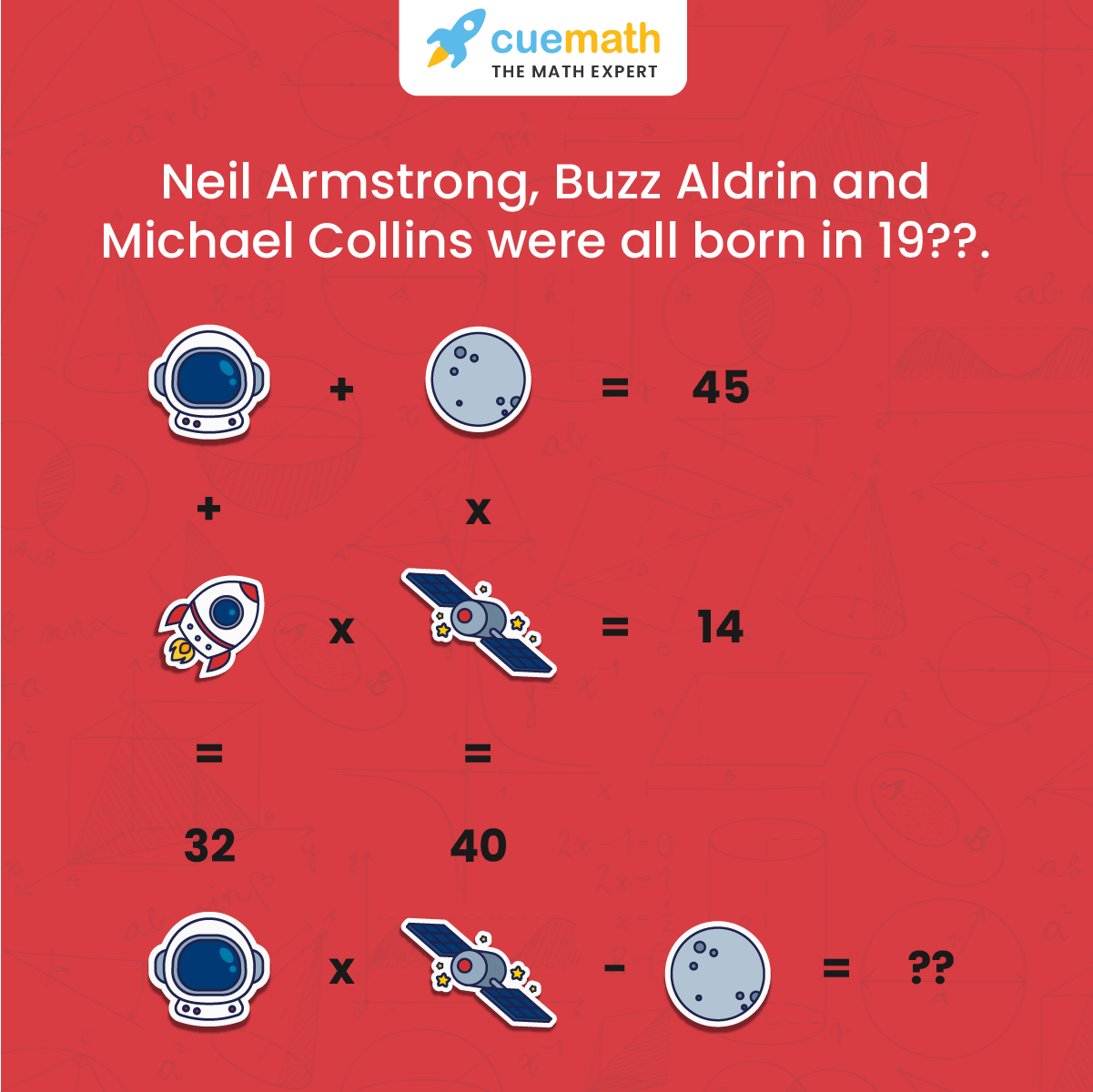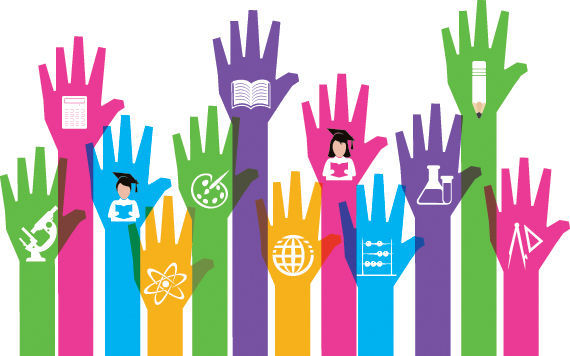Math Puzzles

# Can You Solve This Famous NASA Emoji Puzzle?

2.6k views

 1 Introduction 2 NASA Emoji Puzzle Solution 3 Conclusion 4 About Cuemath 5 FAQs 6 External References

## Introduction

NASA’s STEM engagement account on Twitter celebrated the 50th-anniversary moon landing by posting an interesting puzzle.

Reaching the moon doesn't have to be that hard. Can you solve this math problem, adapted from NASA's own STEM Engagement program?## Famous NASA Emoji Puzzle

Challenge your children to solve these emoji-themed Maths puzzles. Emoji math uses icons and popular emojis in place of numbers, letters, and variables in a math problem. The Famous NASA Emoji Puzzle is mentioned below in the Downloadable PDF.

## NASA Emoji Puzzle Solutions

OK! So, now let’s get down to solving this puzzle. And we have two ways of going about it – a visual method; and a purely algebraic method. Let’s start with the visual one!

### Method #1: Visual MethodNow, this image can be redrawn as (focus on row 2):Further (focus on column 1):Now on replacing Moon we get the following equation, and hence the value of the MoonMoon (m) = 20

Further (focus on column 2):Thus, all of them were born in 1930.

And now let’s do it the algebra way!

### Method #2: Algebraic Method

Let’s assume the values are:

a is the value of the astronaut

m is the value of the moon

r  is the value of the rocket

e is the value of the satellite

Now, let’s form the equation using the puzzle information:

a + m = 45

r x e = 14

a + r = 32

m x e = 40

Using the last equation:

m = 40/e

Putting this into the first equation:

a + 40/e = 45

a = 45-(40/e)

Putting this in the third equation:

45-(40/e) + r = 32

r = 32 – [ 45-(40/e)]

Putting this in the third equation:

{32 – [ 45-(40/e)]} x e = 14

32e – 45e + 40 = 14

45e – 32e = 40-14

13e = 26

e = 2

Therefore,

m = 40/e = 40/2 = 20

a = 45 – m = 45 – 20 = 25

Therefore,

(a x e) – m = (25 x 2) – 20 = 50-20 = 30

Which method did you find to be more interesting? Do let us know in the comment section!

At Cuemath, we teach children to think about more than one way of solving math problems. Also, a Cuemath student gains an advantage in competitive exams as they are trained to use visual problem-solving methods, which yield results in a much shorter period of time.

You can check out all Cuemath’s Puzzles here.

Also, try our exclusive Puzzle Time series: Puzzle Time

Cuemath students enjoy Cuemath’s Puzzle Cards that are curated keeping in mind that age is no bar to enjoy them!

## Conclusion

Innovation leads to new products and processes that sustain our economy. This innovation and science literacy depends on a solid knowledge base in the STEM areas. It is clear that most jobs of the future will require a basic understanding of math and science.Science, technology, engineering and mathematics workers play a key role in the sustained growth and stability of the world economy and are a critical component to helping the world win the future.

STEM education creates critical thinkers, increases science literacy, and enables the next generation of innovators.

Cuemath, a student-friendly mathematics and coding platform, conducts regular Online Live Classes for academics and skill-development and their Mental Math App, on both iOS and Android, is a one-stop solution for kids to develop multiple skills. You can book a Free Class here and know more about the pricing and fees from Cuemath fee for all grades.

## 1. What is STEM Education?

STEM is a curriculum based on the idea of educating students in four specific disciplines — science, technology, engineering and mathematics — in an interdisciplinary and applied approach.

Rather than teach the four disciplines as separate and discrete subjects, STEM integrates them into a cohesive learning paradigm based on real-world applications.

## 2. What is NASA's STEM Engagement Program?

NASA STEM Engagement strives to increase K-12 involvement in NASA projects, enhance higher education, support underrepresented communities, strengthen online education, and boost NASA's contribution to informal education.

The intended outcome is a generation prepared to code, calculate, design, and discover its way to a new era of world innovation.

## External References

Related Articles
Award-winning math curriculum, FREE for a year